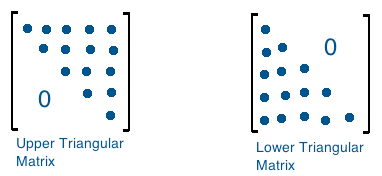# How to Print Lower Triangle Pattern using Python3?

In this Python tutorial, we will learn how to print lower triangle pattern in Python. Let’s see how to print lower triangle pattern of matrix as well as we will print lower triangle star pattern in Python.

## Lower Triangle of the Matrix in Python:

Lower triangle of a matrix consists of diagonal elements and the elements below the diagonal of the matrix.

Let us consider A as 3X3 matrix.

A = {[1 2 3],[4 5 6],[7 8 9]}

And it’s lower triangular matrix is A = {[1 0 0],[4 5 0],[7 8 9]}

i.e.,

```1 2 3            1 0 0
4 5 6   ----->   4 5 0
7 8 9            7 8 9```For more, read from here: https://en.wikipedia.org/wiki/Triangular_matrix

To print a lower matrix pattern here is the code snippet:

PROGRAM:

```n=input("Enter a Symbol of your choice:")
rows=int(input("Enter the no. of rows you wish to execute the pattern:")) #input no. of rows
#print the pattern
for i in range(rows):
for j in range(1,i+1):
print(n,end=" ")
print()```

OUTPUT 1: Print lower triangle hash pattern in Python

```Enter a Symbol of your choice:#
Enter the no. of rows you wish to execute the pattern:10

#
# #
# # #
# # # #
# # # # #
# # # # # #
# # # # # # #
# # # # # # # #
# # # # # # # # #
# # # # # # # # # #
```

OUTPUT 2: Print lower triangle star pattern in Python

```Enter a Symbol of your choice:*
Enter the no. of rows you wish to execute the pattern:8

*
* *
* * *
* * * *
* * * * *
* * * * * *
* * * * * * *
* * * * * * * *```